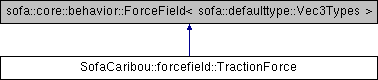Caribou
SofaCaribou::forcefield::TractionForce Class Reference

## Detailed Description

Defines a traction (tractive force) field.

A traction is a force applied to a surface region (planar discretization such as triangle or quad elements). It does not take into account the surface normal, but instead follows a force direction explicitly defined in the input parameters of the component.

This component allows to apply the total tractive force from a set of smaller load increments following a linear slope until the total load is reach, or apply all the load at once.

Template Parameters
 DataTypes The datatype of the coordinates/derivatives vectors (3D float vector, 3D double vector, 2D float vector or 2D double vector).

`#include <TractionForce.h>`

Inheritance diagram for SofaCaribou::forcefield::TractionForce:## Public Types

typedef sofa::defaulttype::Vec3Types DataTypes

typedef DataTypes::VecCoord VecCoord

typedef DataTypes::VecDeriv VecDeriv

typedef DataTypes::Coord Coord

typedef DataTypes::Deriv Deriv

typedef Coord::value_type Real

typedef sofa::defaulttype::Mat< 3, 3, Real > Mat33

template<int nRows, int Options = 0>
using Vector = Eigen::Matrix< Real, nRows, 1, Options >

template<int nRows>
using MapVector = Eigen::Map< const Vector< nRows, Eigen::ColMajor > >

using Triange = TriangleSetTopologyContainer::Triangle

## Public Member Functions

SOFA_CLASS (TractionForce, SOFA_TEMPLATE(sofa::core::behavior::ForceField, sofa::defaulttype::Vec3Types))

void init () override

void reset () override

void addForce (const sofa::core::MechanicalParams *mparams, Data< VecDeriv > &d_f, const Data< VecCoord > &d_x, const Data< VecDeriv > &d_v) override

void addDForce (const sofa::core::MechanicalParams *, Data< VecDeriv > &, const Data< VecDeriv > &) override

void addKToMatrix (sofa::defaulttype::BaseMatrix *, SReal, unsigned int &) override

void draw (const sofa::core::visual::VisualParams *vparams) override

void handleEvent (sofa::core::objectmodel::Event *event) override

SReal getPotentialEnergy (const sofa::core::MechanicalParams *, const Data< VecDeriv > &) const override

Increment the traction load by an increment of traction_increment (vector of tractive force per unit area).

## Public Attributes

Data< Deriv > d_traction
Tractive force per unit area.

Data< sofa::helper::vector< Triange > > d_triangles
List of triangles (ex: [t1p1 t1p2 t1p3 t2p1 t2p2 t2p3 ...])

List of quads (ex: [q1p1 q1p2 q1p3 q1p4 q2p1 q2p2 q2p3 ...])

Data< Real > d_slope
Slope of force increment, the resulting traction will be p^t = p^{t-1} + p*slope. If slope = 0, the force will be constant.

Mechanical state that contains the triangle positions.

Data< unsigned int > d_number_of_steps_before_increment
Number of steps to wait before a load increment. This can be used to simulate a Newton-Raphson solver.

Data< bool > d_draw_faces
Draw the faces on which the traction will be applied.

Data< VecDeriv > d_nodal_forces

Tractive force for each nodes of the surface on which we are applying the traction. More...